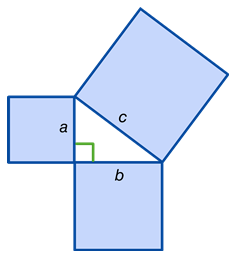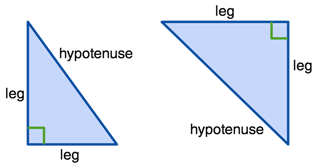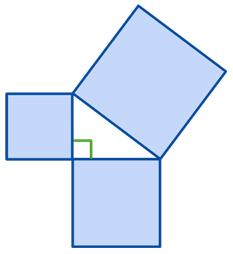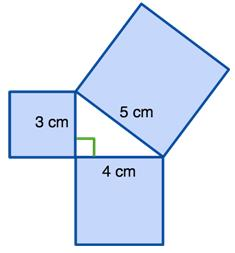Courses

# Pythagorean Theorem Mathematics Notes | EduRev

## Mathematics : Pythagorean Theorem Mathematics Notes | EduRev

The document Pythagorean Theorem Mathematics Notes | EduRev is a part of the Mathematics Course Additional Topics for IIT JAM Mathematics.
All you need of Mathematics at this link: Mathematics

Pythagorean Theorem: a2+b= c2

In a right triangle, the sum of the squares of the two legs equals the square of the hypotenuse.• Legs (a and b): the sides of the triangle adjacent to the right angle. They don't need to be the same length in order for this theorem to work.
• Hypotenuse (c): the side of the triangle opposite the right angle which, conveniently, is always the longest side.So, let's break this down. If you square each side of the triangle, the sum of the areas of the two legs squared is equal to the hypotenuse squared.Here you can see it with numbers:The area of the two smaller squares is (3 × 3 = 9 cm2) and (4 × 4 = 16 cm2).
The area of the larger square is equal to (5 × 5 = 25 cm2).
If you add the two smaller areas together, you get the area of the square of the hypotenuse (9 + 16 = 25 cm2).

Offer running on EduRev: Apply code STAYHOME200 to get INR 200 off on our premium plan EduRev Infinity!

40 docs

,

,

,

,

,

,

,

,

,

,

,

,

,

,

,

,

,

,

,

,

,

;